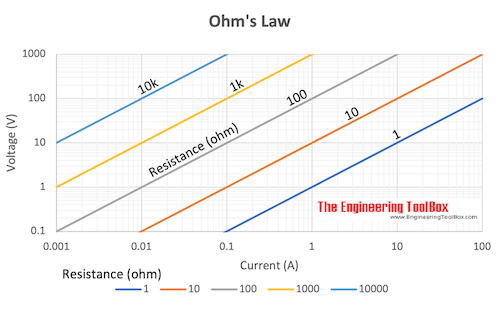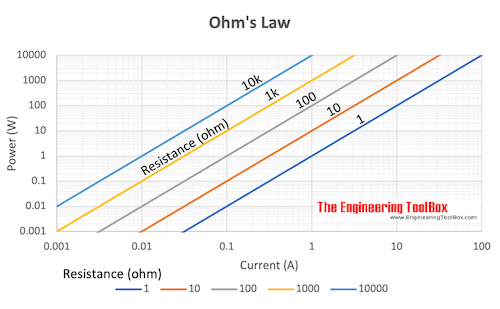Engineering ToolBox - Resources, Tools and Basic Information for Engineering and Design of Technical Applications!

# Electrical Formulas

## Commonly used electrical formulas like Ohms Law and more.

Common electrical units used in formulas and equations are:

• Volt - unit of electrical potential or motive force - potential is required to send one ampere of current through one ohm of resistance
• Ohm - unit of resistance - one ohm is the resistance offered to the passage of one ampere when impelled by one volt
• Ampere - units of current - one ampere is the current which one volt can send through a resistance of one ohm
• Watt - unit of electrical energy or power - one watt is the product of one ampere and one volt - one ampere of current flowing under the force of one volt gives one watt of energy
• Volt Ampere - product of volts and amperes as shown by a voltmeter and ammeter - in direct current systems the volt ampere is the same as watts or the energy delivered - in alternating current systems - the volts and amperes may or may not be 100% synchronous - when synchronous the volt amperes equals the watts on a wattmeter - when not synchronous volt amperes exceed watts - reactive power
• kiloVolt Ampere - one kilovolt ampere - kVA - is equal to 1000 volt amperes
• Power Factor - ratio of watts to volt amperes

### Electrical Potential - Ohm's Law

Ohm's law can be expressed as:

U = R I                        (1a)

U = P / I                      (1b)

U = (P R)1/2                (1c)Download and print Ohm's Law

### Electric Current - Ohm's Law

I = U / R                      (2a)

I = P / U                      (2b)

I = (P / R)1/2               (2c)

### Electric Resistance - Ohm's Law

R = U / I                     (3a)

R = U2/ P                    (3b)

R = P / I2                    (3c)

### Example - Ohm's law

A 12 volt battery supplies power to a resistance of 18 ohms.

I = (12 V) / (18 Ω)

= 0.67 (A)

### Electric Power

P = U I                      (4a)

P = R I2                    (4b)

P = U2/ R                  (4c)

where

P = power (watts, W, J/s)

U = voltage (volts, V)

I = current (amperes, A)

R = resistance (ohms, Ω)Download and print Ohm's Law

### Electric Energy

Electric energy is power multiplied with time:

W = P t                       (5)

where

W = energy (Ws, J)

t = time (s)

Alternative - power can be expressed

P = W / t                     (5b)

Power is consumption of energy by consumption of time.

#### Example - Energy lost in a Resistor

A 12 V battery is connected in series with a resistance of 50 ohm. The power consumed in the resistor can be calculated as

P = (12 V)2 / (50 ohm)

= 2.9 W

The energy dissipated in 60 seconds can be calculated

W = (2.9 W) (60 s)

= 174 Ws, J

= 0.174 kWs

= 4.8 10-5 kWh

#### Example - Electric Stove

An electric stove consumes 5 MJ of energy from a 230 V power supply when turned on in 60 minutes.

The power rating - energy per unit time - of the stove can be calculated as

P = (5 MJ) (106 J/MJ) / ((60 min) (60 s/min))

= 1389 W

= 1.39 kW

The current can be calculated

I = (1389 W) / (230 V)

= 6 ampere

### Electrical Motors

#### Electrical Motor Efficiency

μ = 746 Php / Pinput_w                 (6)

where

μ = efficiency

Php = output horsepower (hp)

Pinput_w = input electrical power (watts)

or alternatively

μ = 746 Php / (1.732 V I PF)                      (6b)

#### Electrical Motor - Power

P3-phase = (U I PF 1.732) / 1,000                    (7)

where

P3-phase = electrical power 3-phase motor (kW)

PF = power factor electrical motor

#### Electrical Motor - Amps

I3-phase = (746 Php) / (1.732 V μ PF)                  (8)

where

I3-phase = electrical current 3-phase motor (amps)

PF = power factor electrical motor

## Related Topics

• ### Electrical

Electrical units, amps and electrical wiring, wire gauge and AWG, electrical formulas and motors.

## Related Documents

• ### 12 Volt - Maximum Wire Length vs. Current

Maximum length of copper wire with 2% voltage drop.
• ### Aluminum Conductor Characteristics

Characteristics of all-aluminum conductors (AAC).
• ### Copper and Aluminum Wire - Max Amps vs. Gauge

Maximum current in copper and aluminum wire.
• ### Copper Wire - Electrical Resistance vs. Gauge

Gauge, weight, circular mils and electrical resistance in copper wire.
• ### Electric Circuit Diagram - Drawing Template

Online shareable electric circuit diagram.
• ### Electrical Equipment - Power Consumption

Typical power consumption and running time for common electrical equipment.
• ### Electrical Units

Definition of common electrical units - like Ampere, Volt, Ohm, Siemens.
• ### Electrical Vehicle Charging - Power vs. Voltage and Amps

EV Charging - AC vs. DC, single phase vs. three phase and power vs. voltage and amps.
• ### Electrical Voltage and Frequency Standards by Country

Typical voltages and frequencies used for domestic appliances in different countries.
• ### Electromotive Force - e.m.f

Change in electrical potential between two points.
• ### Electrotechnical Abbreviations

Abbreviations according the International Electrotechnical Commission (IEC).

Specific gravity and charge of lead acid batteries - temperature and efficiency.
• ### Ohm's Law

The relation between voltage, current and electrical resistance.
• ### Relative vs. Absolute Voltage

Electric circuits and voltage at any point.
• ### Resistance vs. Conductance

The reciprocal of electrical resistance is conductance.
• ### Resistivity and Conductivity - Temperature Coefficients Common Materials

Resistivity, conductivity and temperature coefficients for common materials like silver, gold, platinum, iron and more..
• ### Resistors - Color Codes Calculator

Color codes for fixed resistors - values and tolerances - online calculator.
• ### Single and Three Phase AC - Electric Current vs. Power

Convert between single phase (120, 240 and 480 Voltage) and three phase (240 and 480 Voltage).
• ### Single Phase Power Equations

Power equations for single phased electrical systems.
• ### Three-Phase Power - Equations

Electrical 3-phase equations.

## Engineering ToolBox - SketchUp Extension - Online 3D modeling!

Add standard and customized parametric components - like flange beams, lumbers, piping, stairs and more - to your Sketchup model with the Engineering ToolBox - SketchUp Extension - enabled for use with older versions of the amazing SketchUp Make and the newer "up to date" SketchUp Pro . Add the Engineering ToolBox extension to your SketchUp Make/Pro from the Extension Warehouse !

We don't collect information from our users. More about

## Citation

• The Engineering ToolBox (2003). Electrical Formulas. [online] Available at: https://www.engineeringtoolbox.com/electrical-formulas-d_455.html [Accessed Day Month Year].

Modify the access date according your visit.

9.29.12